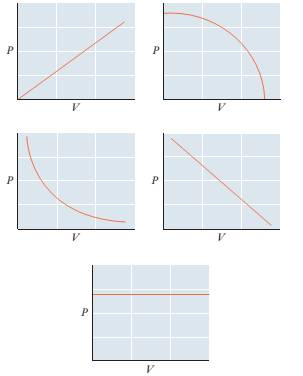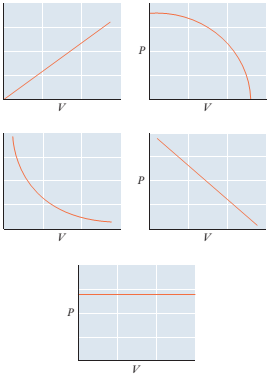Chapter 5, Problem 5.84PAEChemistry for Engineering Students

4th Edition
Lawrence S. Brown + 1 other
ISBN: 9781337398909Chemistry for Engineering Students

4th Edition
Lawrence S. Brown + 1 other
ISBN: 9781337398909
Textbook Problem

84 Consider a sample of an ideal gas with n and T held constant. Which of the graphs below represents the proper relationship between P and V? How would the graph differ for a sample with a larger number of moles?Interpretation Introduction

Interpretation:

Consider a sample of an ideal gas with n and T held constant. The graph representing the proper relationship between P and V needs to be identified. The difference of this from graph with a larger number of moles needs to be explained.Concept introduction:

An ideal gas which is known as the perfect gas is a gas whose volume V, Pressure P and temperature T are related through the ideal gas laws: -

PV = nRT

Here,

n = number of moles of the gas

R = ideal gas constant

T = Temperature

P = Pressure

Ideal gases are described as the molecules which have negligible size but have an average molar kinetic energy which is dependent on the temperature. When temperature is low most of the gases behave like ideal gases and the ideal gas law might be applied to them.

Explanation

Constant temperature means an isothermal process.

PV=nRT

This is the ideal gas equation.

Now, nR is constant.

T is also constant.

So, the equation becomes:

PV=(constant)

Or,

PV=C

In simple terms the product of P and V is constant

Still sussing out bartleby?

Check out a sample textbook solution.

See a sample solution

The Solution to Your Study Problems

Bartleby provides explanations to thousands of textbook problems written by our experts, many with advanced degrees!

Get Started

The freezing point of water is _________ K.

Introductory Chemistry: A Foundation

Behavior change is a process that takes place in stages. T F

Nutrition: Concepts and Controversies - Standalone book (MindTap Course List)

Why have astronomers added modern constellations to the sky?

Horizons: Exploring the Universe (MindTap Course List)